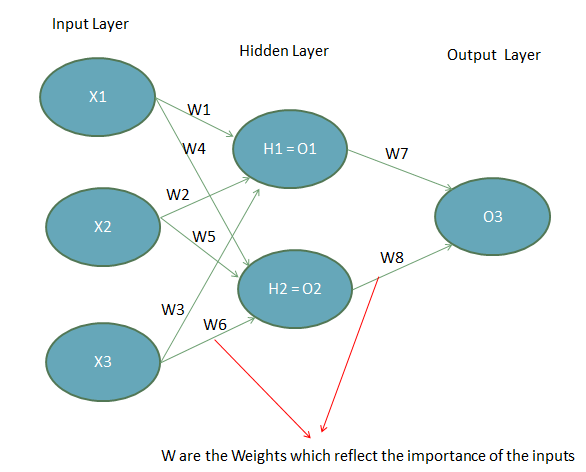# Neural Networks Overview

Neural networks is an advanced technique which is within the field of Deep Learning. As we know, machine learning involves working with algorithms that try to predict a target variable or segment data to find relevant patterns without human intervention.

In contrast, in deep learning architecture there is more than one layer of these algorithms and these systems represent a network of algorithms that do not necessarily need labeled data to make predictions.  Below we can visualize the main components of a neural network.

Neural Network ArchitectureA neural network is composed of input layers, hidden layers and output layers. The input layers are the beginning of the workflow in a neural network and receive information about the initial data or the features of a dataset.

Hidden layers identify the important information from the inputs, leaving out the redundant information. They convert the network more efficiently and pass the important patterns of data to the next layer which is the output layer.

In the middle of the hidden layer and the output layer, the network has an activation function. This activation function transforms the inputs in the desired output and can take many forms such as a sigmoid function (as in the binary classification of logistic regression) or other types of functions (RELU, Tanh, softmax among others that are beyond the scope of this post).

The goal of the activation function is to capture non-linear relationships between the inputs and on the other hand it helps to convert the inputs into more useful output.

The figure above shows a network architecture called “feed-forward network” as the inputs’ signals flow in only one direction (from inputs to outputs). There are other types of flows such as back propagations, where the data goes back along the neural networks and inspect every connection to check how the output would behave according to a change in the weight. The key of the model is to find the optimal weights values of W that minimize the prediction error. Just like other machine learning algorithms, the neural networks also have a loss function that will be minimized.

One common optimization algorithm to minimize the loss function that is used in neural networks is called “gradient descent” that works on multiple iterations trying to find the weights that returns the less value of the error term.

The inner steps of gradient descent algorithm are the following:

1. Assign random values for the weights and pass them as parameters of the function
2. Calculate the change in Sum Squared Error (SSE) when the weights are changed by small amounts from their original random values
3. Adjust the weights to reduce the impact of the prediction errors
4. Use the new weights for predictions and to calculate the new SSE
5. Repeat steps 2 and 3 until the adjustments in weights don’t significantly reduce the error term.

There are some important advantages of neural networks that are pointed below:

• They are suitable to represent and deal with not-linear relationships in the data.
• These models are better choices to avoid overfitting on the model. After learning from their initial inputs and the relationship of the factors, they are able to generalize and predict on unseen data.
• They do not impose restrictions on the input variables such as some distribution pattern of the data.
• They are able to model heteroscedasticity data with high volatility and non-constant variance. This represents a breakthrough in the financial time series prediction, where the data has high and time dependent variance. Heteroscedasticity means that the variability or variance of the time series is not constant over time. The opposite is called homoscedasticity.

Due to their ability to model complex relationships and understand underlying factors of the data, these models are a good tool to forecast stock prices. Recent advances in the field are the usage of Long Short Term Memory (LSTM) models and Recurrent Neural Network for forecasting.